Graph all the things

analyzing all the things you forgot to wonder about

# Wordle

2022-01-23

EDIT: I have been informed that I was using a clone of the original Wordle. The clone modified the word lists somewhat and introduced non-5-letter word functionality that was not present in the original. Redoing the analysis on the original's word lists, "SOARE" is the best mean word, and "ARISE" and "RAISE" are tied for the best minimaxing words (getting down to at most 168 possible answers). "RAISE" is also 3rd place in terms of entropy, with 4.074 vs "SOARE"'s 4.080, so I'd probably use "RAISE" in general. However, it looks like the word lists are gradually changing as well, so these results may vary over time.

The rest of this post remains unaltered and still contains interesting material, even if you don't care about the clone.

### Prelude

The best starting 5-letter guess in Wordle is probably "CRATE" or "ROATE", depending on how you measure. For 4-to-11-letter words, I have a table below with the best guesses. I know some of the best words don't seem like words, but they're in the Wordle vocabulary.

If you haven't played it, go try out Wordle for a bit. It's like Hangman but more interesting. It has a better explanation of the rules than I can render.

I made a shoddy AI to play Wordle and learned a few things myself. For instance, the game gets much easier as word length increases; the number of words in the answer list stays about the same, but you get much more information from each guess. Also, I found a slight issue in their code and reverse-engineered the random seed to surface it - try this puzzle with word length set to 8 (go to the bottom of this article to see an explanation).

### Goals

Here are two goals one might have in Wordle:

1. Minimize the worst-case number of guesses you use per puzzle, i.e. you want to almost guarantee solving the puzzle in 6 guesses.
2. Minimize the mean number of guesses you use per puzzle, i.e. you want to use fewer guesses on average than someone else.

I'll call goal 1 the minimax goal and goal 2 the mean goal.

You may have already heard of minimaxing - it's a strategy where you choose each guess to minimize the worst outcome your opponent could cause you. For instance, a minimaxing chess player A would consider every legal move and choose the one such that the best follow-up move their opponent B could make leaves A in as strong a possible as possible. In Wordle, you can view the randomness of the game as the opponent. There are 953 possible 5-letter word answers, and if you start with a bad guess like "FUFFY", the worst outcome is all gray squares, leaving you with a whopping 641 possibilities remaining (fun fact: "FUFFY" is the worst minimax guess, narrowly losing to "YUKKY" and "FUZZY"). On the other hand, if you start with "ROATE", no matter what outcome the game gives you, there are at most 41 possibilities remaining (fun fact: for "ROATE", the worst outcome is not all gray squares, but a yellow square on "A").

In order to solve the puzzle quickly in the average case instead of worst case, the mean goal maximizes the outcome distribution's entropy. To understand the outcome distribution's entropy, consider playing an-letter word. There arepossible outcomes - gray, yellow, or green for each tile. There is a probabilityfor each outcomeproportional to the number of words in the answer set that would give that outcome. The entropy of the outcome distribution is computed as the average log of theover the distribution of outcomes, orMaximizing entropy gets us to the answer approximately as fast as possible on average because the depth of a tree tends to be logarithmic with the number of nodes it contains. In other words, if we have 1,000 words in the answer set and each guess we make narrows us down to 1/10 of those on average, we should expect to make aboutguesses before knowing what the answer is. When we maximize entropy, we choose the guess such that on average, after observing the outcome, we have a small logarithm of the number of possibilities remaining. This small logarithm roughly corresponds to the number of following guesses it will take us to solve the puzzle.

### Shoddy AI

I wrote a shoddy AI to play Wordle.

To do so, I downloaded the Wordle vocabulary (a JSON blob network response). As I later discovered, there is also a list of possible answers (a subset of the vocabulary) hard-coded into the site, and friggin "CUPOLA" is in it! Plus the word "MARCH" is in the answer list twice, so it's twice as likely as the other 5-letter words. And there's one other quirk I explain at the bottom of this post. Anyway, I used the vocabulary and answer lists in making the AI.

The AI is just a script. It asks you to input the guesses you've made and their outcomes, and it tells you the best word to guess according to either the mean goal or minimax goal. It does this by crunching through all combinations of words in the vocabulary and still valid words in the answer set, evaluating the outcome each answer would give. After you input a word and its outcome, the script then eliminates all the answers that don't agree. Here's an example game I played and how I entered it in the program (filling in the > what was the result? prompts):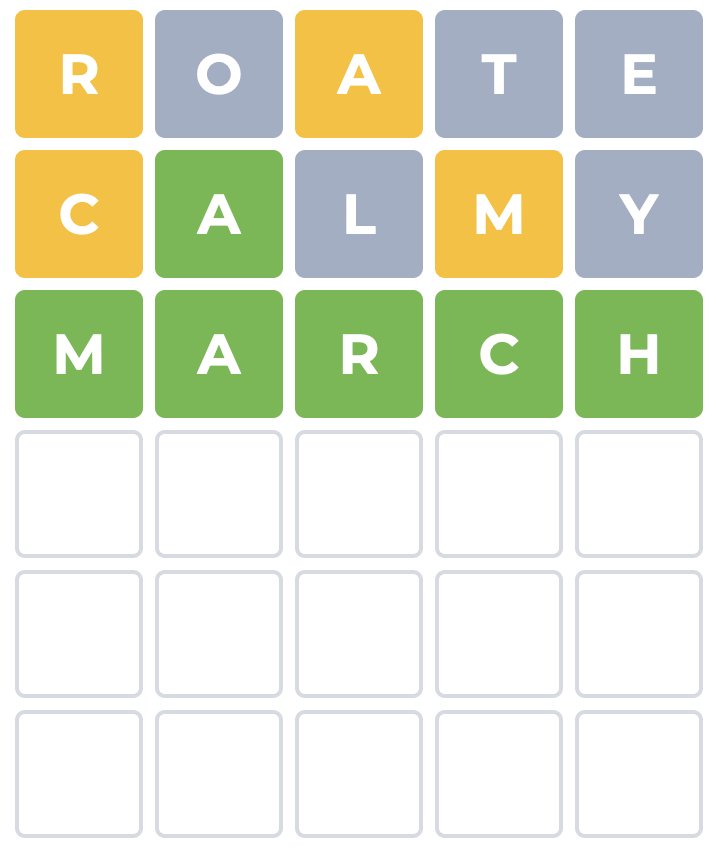% python3 shoddy_ai.py 5 0 MINIMAX QUIET
running goal='MINIMAX' for n=5 letters
953 remaining possibilities
computing best words...
rank=0 word='roate' score=41 is_possible_answer=False
rank=1 word='soare' score=52 is_possible_answer=False
rank=2 word='aeros' score=55 is_possible_answer=False
rank=3 word='arose' score=55 is_possible_answer=False
rank=4 word='irate' score=56 is_possible_answer=False
> what was the result? r1o0a1t0e0
15 remaining possibilities
computing best words...
rank=0 word='calmy' score=2 is_possible_answer=False
rank=1 word='calps' score=2 is_possible_answer=False
rank=2 word='calyx' score=2 is_possible_answer=False
rank=3 word='campi' score=2 is_possible_answer=False
rank=4 word='campy' score=2 is_possible_answer=False
> what was the result? c1a2l0m1y0
1 remaining possibilities
the word: march

Here I just input the color I got for each letter by following it with a 0 (gray), 1 (yellow), or 2 (green). So r1o0a1t0e0 describes the outcome I got for "ROATE".

The AI doesn't play quite optimally - it only optimizes its objective over a single guess, rather than the whole game. But for the most part, I can't tell the difference. When I play, I also greedily narrow the possibilities.

### Optimal Starting Guesses

People have been speculating about the best starter word. My script is just one methodology. It's not computationally possible to solve the optimal starting guess exactly, for either of these goals. But this little AI does provide its own take on the matter, depending on your goal:

# of letters Goal Optimal Guess Minimax Score Entropy Score
4 minimax "OLEA" 107 2.74
4 mean "TALE" 150 3.14
5 minimax "ROATE" 41 4.11
5 mean "CRATE" 60 4.15
6 minimax "TAILOR" 29 4.84
6 mean "CARNET" 46 5.05
7 minimax "SALTIER" 14 5.75
7 mean "SALTIRE" 17 5.81
8 minimax "COTELINE" 7 6.08
8 mean "CAROTINS" 8 6.15
9 minimax "ACROSPIRE" 5 6.07
9 mean "ANORETICS" 5 6.29
10 minimax "ALACTRITIES" 4 5.87
10 mean "SUPERCLEAN" 5 5.97
11 minimax "ADMIRALTIES" 2 5.52
11 mean "IMPERIALISE" 3 5.57

As you can see, the game gets easier with longer words; after the first guess in an 11-letter game, you can narrow down to 2 possible words immediately, whereas in a 4-letter game, you might still have 107 to contend with. I find that the main challenge for longer words as a human is recalling words that fit all the constraints.

### Example Games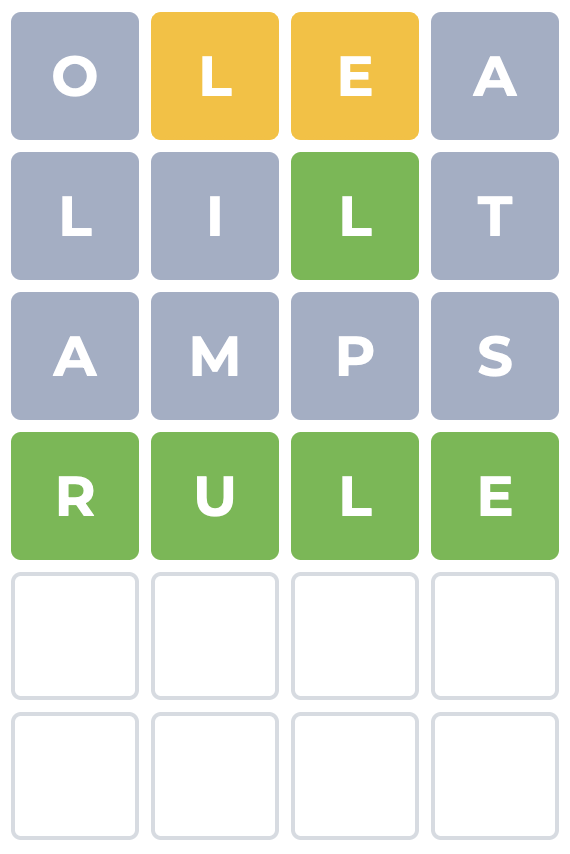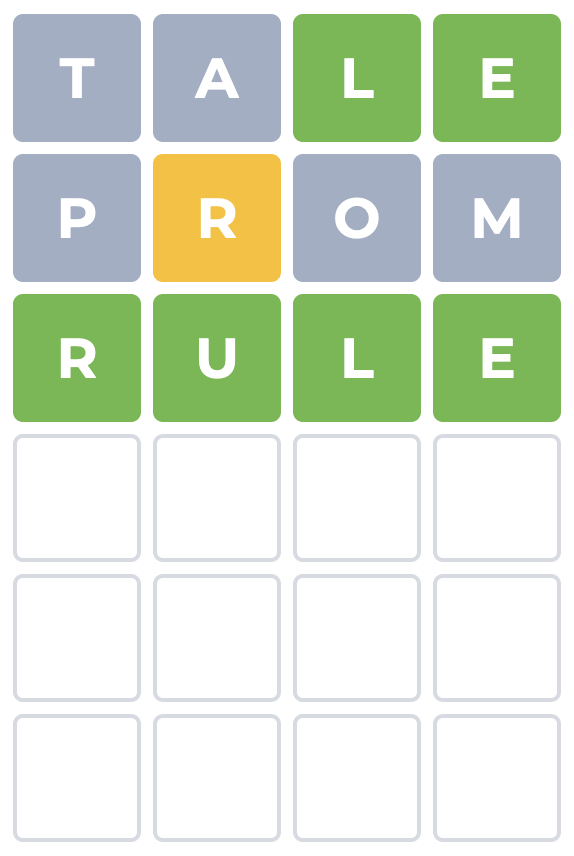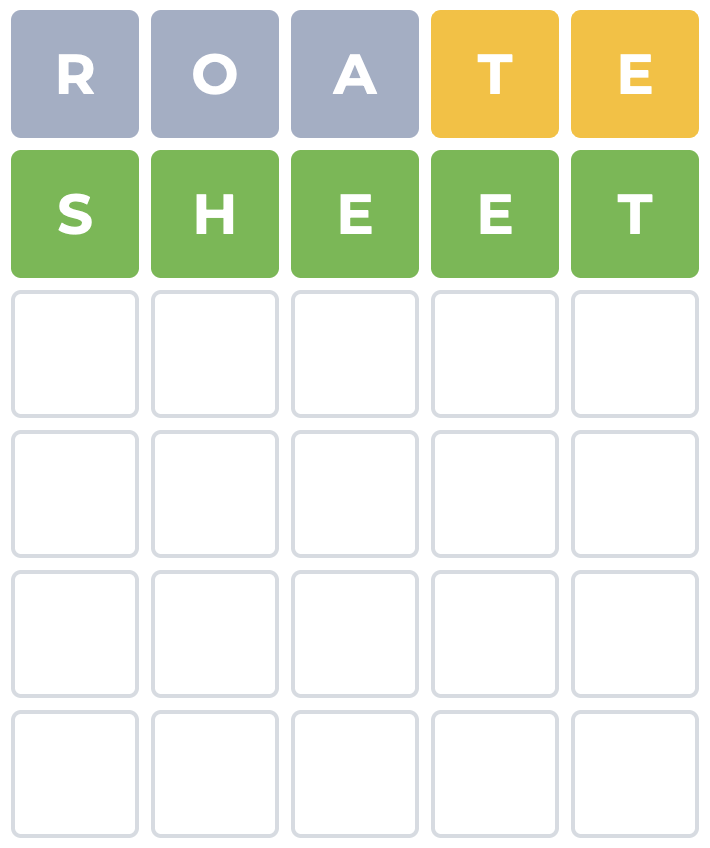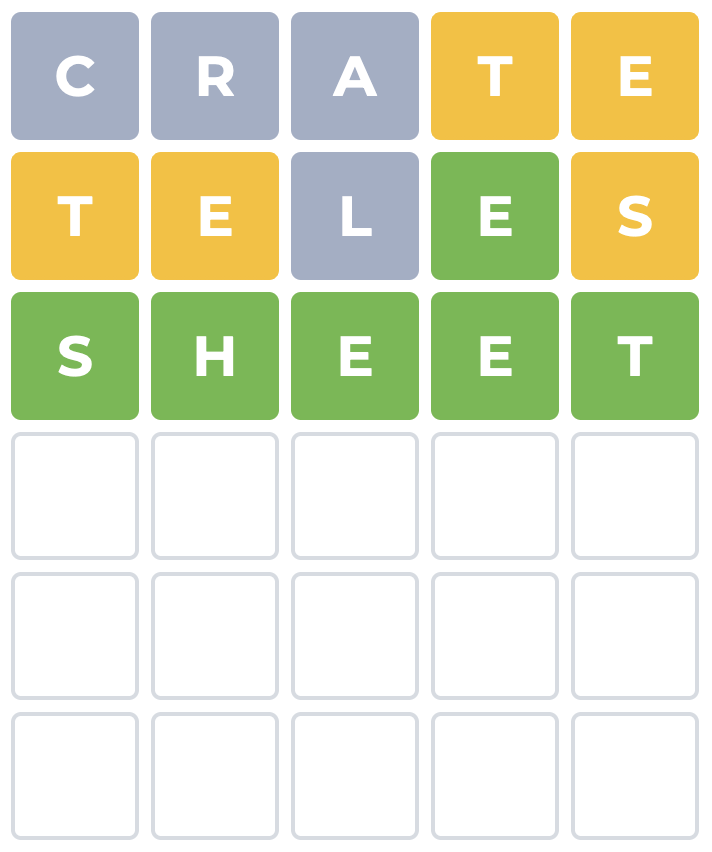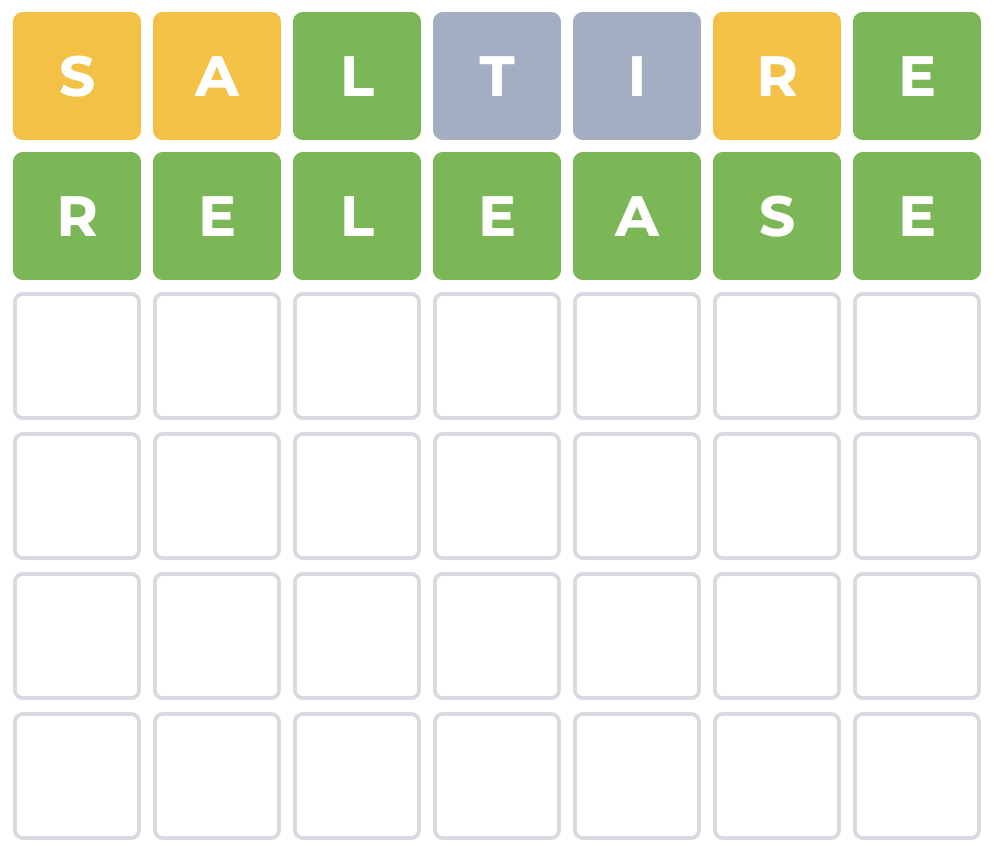### Puzzle 994 Length 8

I alluded to an issue in the Wordle code at the beginning of this article, surfacing on the puzzle with seed 994 and 8 letters. The problem is that the Wordle answer set contains "JALAPEÑO" with the accent character. The game behaves strangely, telling you that there is is no "N", but accepting "JALAPENO" as an answer: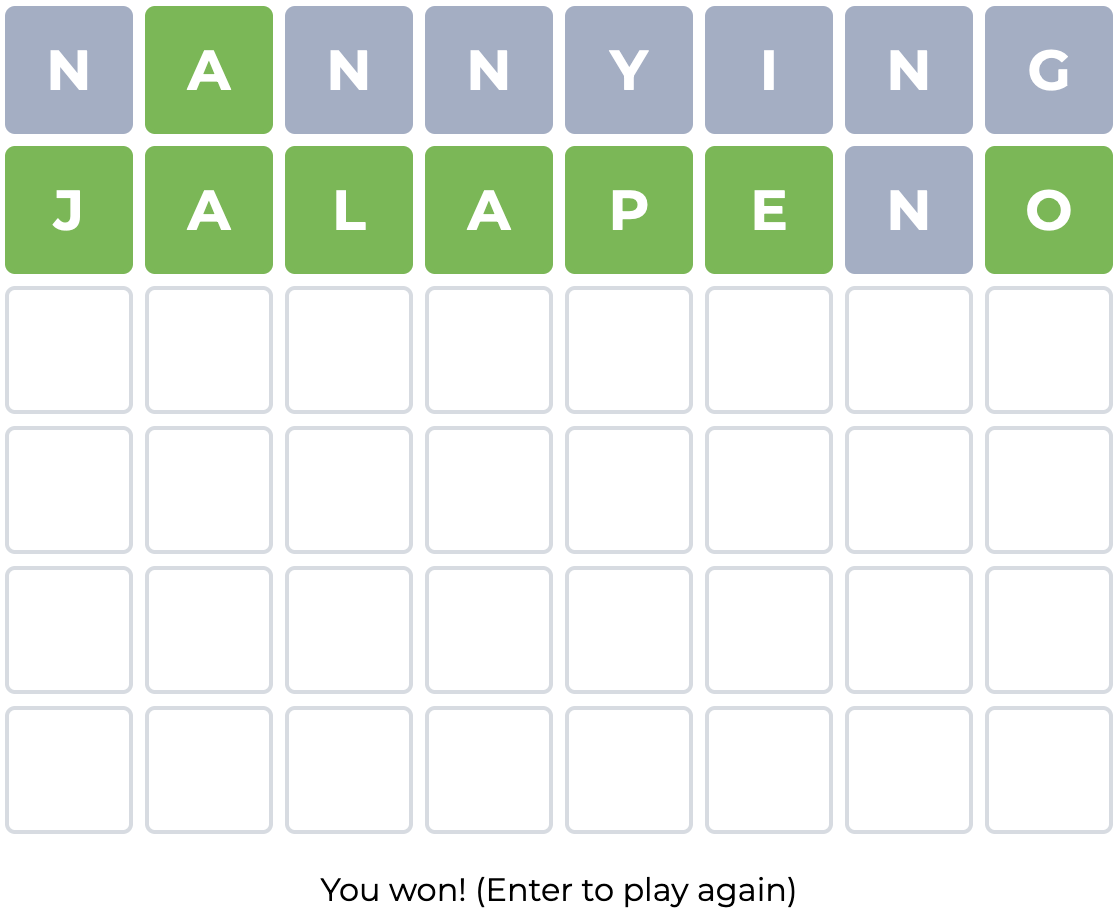I found this example by noticing that the copy-pasted answer list didn't parse as JSON. The "Ñ" was the only non-ascii character, so I knew it was the only example. To actually play with the word, I had to reverse-engineer a random seed that would select it. I found their source code for seeded random generation - an XORshift algorithm (very similar to one I've written before for this blog) - and simply brute forced it until I found a good seed. It didn't take long because there are only 854 8-letter words in the answer set.